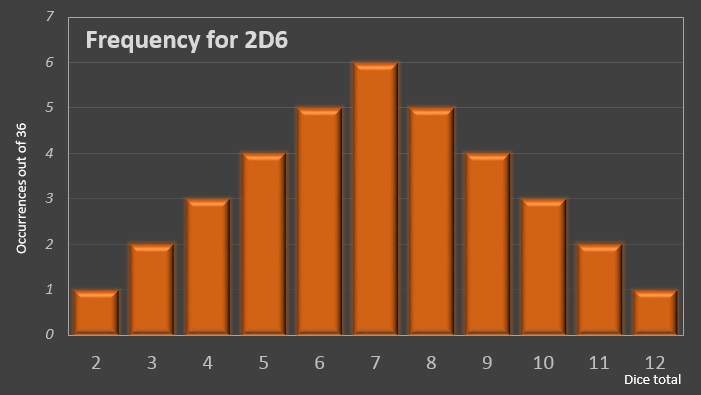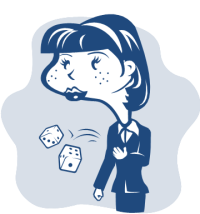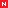﻿ Sicherman Dice# Rolling DiceA standard die has six sides labeled 1-6. Each score is equally likely.If we roll two dice and add their scores together, the total score follows the distribution pattern below.

As any craps player will tell you, the most likely sum of two dice is 7, which can be rolled 6 different ways out of the 36 possible combinations.Here is the breakdown of how these totals are made:

 2 {1,1} 3 {1,2} {2,1} 4 {1,3} {2,2} {3,1} 5 {1,4} {2,3} {3,2} {4,1} 6 {1,5} {2,4} {3,3} {4,2} {5,1} 7 {1,6} {2,5} {3,4} {4,3} {5,2} {6,1} 8 {2,6} {3,5} {4,4} {5,3} {6,2} 9 {3,6} {4,5} {5,4} {6,3} 10 {4,6} {5,5} {6,4} 11 {5,6} {6,5} 12 {6,6}
 Here’s a question for you: Is it possible to label two dice in a different (non-standard) way so that the distribution of their totals follows the same pattern?### Sicherman Dice

If it is possible to do then, obviously, the two alternative dice would need to be different from each other (since in the example above we are rolling two of the same die!)

As it happens, such a pair of dice does exist!

They were first documented as being discovered by a Colonel George Sicherman, of Buffalo, New York in a 1978 article in Scientific American, and since that time have been referred to as Sicherman Dice (and sometimes as "Crazy Dice")

Here are meshes for the dice:

 Dice #1 Dice #2(1,2,2,3,3,4) (1,3,4,5,6,8)

On a regular die, the spots are arranged on the cube such the opposite faces add up to 7. On a Sicherman set, interestingly, it's possible to configure the spots so that, on the first, they add up to 5, and on the second they add up to 9.

Here is how the distributions are made up using the new dice, and you can see there are the correct number of entries per row to match the pair of regular dice. (You'll note that one of the dice contains repeated numbers, and the other has one spot count of eight).

Sicherman Dice Regular Dice
 2 {1,1} 3 {2,1} {2,1} 4 {3,1} {3,1} {1,3} 5 {1,4} {2,3} {2,3} {4,1} 6 {1,5} {2,4} {2,4} {3,3} {3,3} 7 {1,6} {2,5} {2,5} {3,4} {3,4} {4,3} 8 {2,6} {2,6} {3,5} {3,5} {4,4} 9 {1,8} {3,6} {3,6} {4,5} 10 {2,8} {2,8} {4,6} 11 {3,8} {3,8} 12 {4,8}
 2 {1,1} 3 {1,2} {2,1} 4 {1,3} {2,2} {3,1} 5 {1,4} {2,3} {3,2} {4,1} 6 {1,5} {2,4} {3,3} {4,2} {5,1} 7 {1,6} {2,5} {3,4} {4,3} {5,2} {6,1} 8 {2,6} {3,5} {4,4} {5,3} {6,2} 9 {3,6} {4,5} {5,4} {6,3} 10 {4,6} {5,5} {6,4} 11 {5,6} {6,5} 12 {6,6}

Are there any other solutions?

As it happens, there is only one solution to this puzzle (we'll look at a mathematical proof later), with the constraint that each number must be positive. If we relax the rules to allow blank faces (equivalent to allowing a zero), then there are three addtional solutions possible:

 Dice 1a:   0     1     1     2     2     3   Dice 2a:   2     4     5     6     7     9 Dice 1b:   2     3     3     4     4     5   Dice 2b:   0     2     3     4     5     7 Dice 1c:   0     1     2     3     4     5   Dice 2c:   2     3     4     5     6     7

If we go further, and allow negative numbers on the faces, there are an infinite number of solutions. How?, well, since we're adding the two dice together, we can simply "Rob Peter to pay Paul". We can add any arbitrary number to all the faces of one die and subtract the same number from all the faces on the other. When the two dice are added, this number will cancel.

(A1 - n, A2 - n, A3 - n, A4 - n, A5 - n, A6 - n)   and   (B1 + n, B2 + n, B3 + n, B4 + n, B5 + n, B6 + n)

Subtracting any number greater than two, obviously, has the potential to create negative numbers on one of the dice.

Of course, there are two classes of solution if we allow negative numbers. Those that involve addition and subtraction from the base Sicherman solution, and those that add and subtract an arbitrary number from a base set of regular dice.

 Dice 1d:   -1     0     0     1     1     2   Dice 2d:   3     5     6     7     8     10   Adding/Subtracting 2 from Sicherman Dice. Dice 1e:   -1     0     1     2     3     4   Dice 2e:   3     4     5     6     7     8   Adding/Subtracting 2 from two regular dice.

### Do not go to jail …So, is a Sicherman set exactly the same as a pair of regular dice? No! Sure, the sum of spots is the same, and if this is all you use, then go ahead, but many games rely on the concept of rolling doubles. In Monopoly for instance, rolling a double allows you to roll again, and three doubles in a row send you to Jail.

Sicherman Dice roll doubles less often. For a regular pair of dice, doubles occur one time in six, and any face can be rolled as a double. On standard Sicherman dice, doubles only occur four times out of thirty-six, and the only doubles you will see are double-ones, double-threes and double-fours (with the double-threes occuring more often, on average, than the other doubles).

### Proof

To prove that there is only one (positive) solution to the Sicherman Dice problem requires quite a bit of math, and the concept of Generating Polynomials. I'll show a good portion of the work here and reference other sources if you want to get the complete story.

We can represent the features of each die by a polynomial function as a superposition of terms of the form Axn where A is the frequency of occurrence of each face with the value n

For example a standard die D can be represented as:

D = x1 + x2 + x3 + x4 + x5 + x6

To generate all possible outcomes of two similar regular dice we multiply their generating polygons.

D2 = (x1 + x2 + x3 + x4 + x5 + x6)2

D2 = x2 + 2x3 + 3x4 + 4x5 + 5x6 + 6x7 + 5x8 + 4x9 + 3x10 + 2x11 + x12

Here we can see the binomial distribution of terms and coefficients showing the permutations and their frequencies. We can see, for instance, the term 6x7 showing that the answer of seven appears with frequency 6 in the solution.

To find an alternate solution we need to find another way to factorize this equation, which will help us define two alternative polynomials that can be multipled together to obtain the same result. An alternative factorization for D is shown below (feel free to expand it out on a piece of paper to confirm it for yourself!):

D = x (1 + x) (1 - x + x2) (1 + x + x2)

So, an alternate way to write D2 is:

D2 = [ x (1 + x) (1 - x + x2) (1 + x + x2) ] [ x (1 + x) (1 - x + x2) (1 + x + x2) ]

Shuffling the deck-chairs around we can make this:

D2 = [ x (1 + x) (1 + x + x2) ] [ x (1 + x) (1 - x + x2)2 (1 + x + x2) ]

Let's call the collection of terms in the lefthand square bracket P and those in the right Q:

P = x (1 + x) (1 + x + x2)

Q = x (1 + x) (1 - x + x2)2 (1 + x + x2)

Here's where the magic happens, we can now expand out these two terms:

 P = (x + x2) (1 + x + x2) P = x + x2 + x2 + x3 + x3 + x4 P = x + 2x2 + 2x3 + x4 Q = (x + x2) (1 - x + x2)2 (1 + x + x2) Q = (x + x2) (1 - 2x + 3x2 - 2x3 + x4) (1 + x + x2) Q = x + x3 + x4 + x5 + x6 + x8

These generating functions neatly describe the Sicherman Dice solution: [1,2,2,3,3,4] and [1,3,4,5,6,8]

Now, to show that there are no other ways to factorize the equation (subject to our constraints) is a little more complex. If you love math, you can read about it here from this paper. The proof pivots on the constraints that we know that both of the dice need to have the number one on their faces, after all, the only way to generate a sum of two is if there is a one from each die. We know, therefore, that however we split the equation D2 into the two components P and Q that each side must contain x (or some positive multiple of).

### Other interesting dice sets

As hinted at earlier, the rolling of seven in the game of craps is very important. Can we use what we've learned ealier to make to a set of dice that improves the chances of rolling seven?

Yes, we can!

The problem, however, is that non-standard dice are easy to detect.

Dice that have more spots on than six would not even pass a cursory inspection; they just look wrong, and a die that obviously has more than one of the same number similarly looks weird.

However, take a look at this pair:(1,1,2,2,3,3) (4,4,5,5,6,6)When you look at any die at most you can only ever see three sides at the same time. Take a careful look at the dice above. No matter what angle you look at them, they appear to be 'normal' dice. If you don't have an opportunity to pick them up and examine them, or if you don't change your point of view as you look at them (to reveal a previously unseen face), you would be ignorant that they are gimmicked. The dice on the left, is it a regular die, or a gimmick? Let's call these dice Crap Cheating Dice.

Let's look at the distribution of scores that are generated by these crap cheating dice and compare them to the scores that can be obtained by regular dice.

Crap Cheating Dice Regular Dice
 2 3 4 5 {4,1} {4,1} {4,1} {4,1} 6 {4,2} {4,2} {4,2} {4,2} {5,1} {5,1} {5,1} {5,1} 7 {4,3} {4,3} {4,3} {4,3} {5,2} {5,2} {5,2} {5,2} {6,1} {6,1} {6,1} {6,1} 8 {5,3} {5,3} {5,3} {5,3} {6,2} {6,2} {6,2} {6,2} 9 {6,3} {6,3} {6,3} {6,3} 10 11 12
 2 {1,1} 3 {1,2} {2,1} 4 {1,3} {2,2} {3,1} 5 {1,4} {2,3} {3,2} {4,1} 6 {1,5} {2,4} {3,3} {4,2} {5,1} 7 {1,6} {2,5} {3,4} {4,3} {5,2} {6,1} 8 {2,6} {3,5} {4,4} {5,3} {6,2} 9 {3,6} {4,5} {5,4} {6,3} 10 {4,6} {5,5} {6,4} 11 {5,6} {6,5} 12 {6,6}
 Using our crap cheating pair, there are 12 permutations of rolls (out of 36) that will roll a seven. This equates to a 1/3 chance of rolling a seven! Not too shabby! This is a two-fold improvement over a regular pair of dice in which there are only 6 permuations out of 36 which result in a score of seven giving odds of 1/6  WARNING  For the record, I just want to say that attempting to make these dice to cheat, con, or swindle money out of innocent people people is wrong on an ethical level, even before any legal implications are calculated. I'm describing their construction here for educational purposes only.If you like dice articles, you might enjoying reading this article about Intransitive Dice, or this one about Yahtzee. Alternatively, check out other interesting blogarticles.

You can find a complete list of all the articles here.Click here to receive email alerts on new articles.